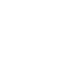### 联系我们

Email：[email protected]

# 许多纺织新手都搞不清的纱线计算公式和换算关系，看了这篇秒懂

### 发布日期：2017-10-20 08:46:46 来源： 点击：（1） 旦尼尔(D)：D=g/L * 9000 其中g为丝线的重量（克），L为丝线的长度（米）
（2） 特克斯（号数）[tex(H)]：tex=g/L * 1000 ，其中g为纱（丝）的重量（克），L为纱（或丝）的长度（米）
（3） 分特克斯(dtex)： dtex=g/L * 10000 ，其中g为丝线的重量（克），L为丝线的长度（米）

（1） 公制支数(N)：N=L / G ，其中G为纱（丝）的重量（克），L为纱（丝）的长度（米）

（2） 英制支数(S)：S=L / (G * 840) ，其中G为丝线的重量（磅），L为丝线的长度（码）

（1） 公制支数(N)与旦尼尔(D)的换算关系：

D=9000/N

（2） 英制支数(S)与旦尼尔(D)的换算关系：

D=5315/S

（3） 分特克斯(dtex)与特克斯(tex)的换算关系：

1 tex=10 dtex

（1） 特克斯(tex)与旦尼尔(D)的换算公式：

tex=D/9

（2） 特克斯(tex)与英制支数(S)的换算公式：tex=K/S（K=590.5）
（3） 特克斯(tex)与公制支数(N)的换算公式：tex=1000/N
（4） 分特克斯(dtex)与旦尼尔(D)的换算公式：dtex=10D/9
（5） 分特克斯(dtex)与英制支数(S)的换算公式：dtex=0.1K/S（K=590.5）
（6） 分特克斯(dtex)与公制支数(N)的换算公式：dtex=100/N

（1） 公制厘米(cm)与英制英寸(inch)的换算公式：

1 inch=2.54 cm

（2） 公制米(m)与英制码(yd)的换算公式：

1码=0.9144m

（3） 绸缎平方米克重(g/m2)与姆米(m/m)的换算公式：

1m/m=4.3056g /m2

（4） 绸缎的实际重量与磅重的换算公式：### 相关标签：

﻿